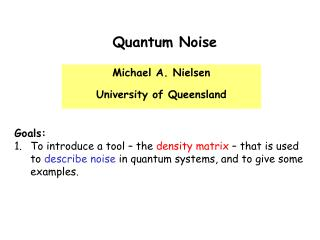DownloadDownload PresentationMichael A. Nielsen University of Queensland

Michael A. Nielsen University of Queensland

Download PresentationMichael A. Nielsen University of Queensland

- - - - - - - - - - - - - - - - - - - - - - - - - - - E N D - - - - - - - - - - - - - - - - - - - - - - - - - - -
Presentation Transcript

1. Quantum Noise Michael A. Nielsen University of Queensland • Goals: • To introduce a tool – the density matrix – that is used to describe noise in quantum systems, and to give some examples.

2. Density matrices Generalization of the quantum state used to describe noisy quantum systems. Terminology: “Density matrix” = “Density operator” Quantum subsystem Ensemble Fundamental point of view

3. What we’re going to do in this lecture, and why we’re doing it Most of the lecture will be spent understanding the density matrix. Unfortunately, that means we’ve got to master arather complex formalism. It might seem a little strange, since the density matrix is never essential for calculations – it’s a mathematical tool, introduced for convenience. Why bother with it? The density matrix seems to be a very deep abstraction – once you’ve mastered the formalism, it becomes far easier to understand many other things, including quantum noise, quantum error-correction, quantum entanglement, and quantum communication.

4. Outer product notation

5. Outer product notation

6. Outer product notation One of the advantages of the outer product notation is that it provides a convenient tool with which to describe projectors, and thus quantum measurements.

7. The trace operation Exercise: Prove that tr(|aihb|) = hb|ai.

8. I. Ensemble point of view Probability of outcome k being in state j Probability of being in state j

9. Qubit example: calculate the density matrix conjugate Density matrix Density matrix is a generalization of state

10. Qubit example: a measurement using density matrix

11. Why work with density matrices? Answer:Simplicity! The quantum state is: ? We know the probabilities of states and we want to find or check the density matrix

12. Dynamics and the density matrix Initial density matrix

13. Dynamics and the density matrix This way, we can calculate a new density matrix from old density matrix and unitary evolution matrix U This is analogous to calculate a new state from old state and unitary evolution matrix U. The new formalism is more powerful since it refers also to mixed states.

14. Single-qubit example: calculating new density matrix by operating with an inverter on old density matrix “Completely mixed state”

15. How the density matrix changes during a measurement

16. Characterizing the density matrix What class of matrices correspond to possible density matrices? Trace of a density matrix is one

17. Summary of the ensemble point of view